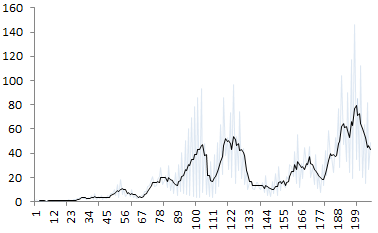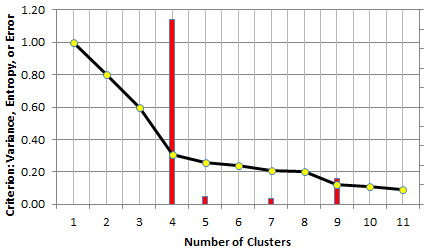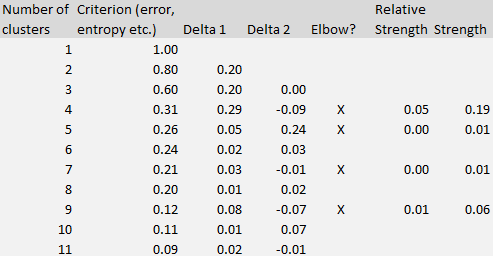# Global AI and Data Science

View Only

## The Elbow Rule

####By Moloy De posted Thu July 02, 2020 08:54 PM

Determining the number of clusters when performing unsupervised clustering is a tricky problem. Many data sets don't exhibit well separated clusters, and two human beings asked to visually tell the number of clusters by looking at a chart, are likely to provide two different answers. Sometimes clusters overlap with each other, and large clusters contain sub-clusters, making a decision not easy.

For instance, how many clusters do you see in the picture below? What is the optimum number of clusters? No one can tell with certainty, not AI, not a human being, not an algorithm.In the above picture, the underlying data suggests that there are three main clusters. But an answer such as 6 or 7, seems equally valid.

A number of empirical approaches have been used to determine the number of clusters in a data set. They usually fit into two categories:

• Model fitting techniques: an example is using a mixture model to fit with your data, and determine the optimum number of components; or use density estimation techniques, and test for the number of modes. Sometimes, the fit is compared with that of a model where observations are uniformly distributed on the entire support domain, thus with no cluster; you may have to estimate the support domain in question, and assume that it is not made of disjoint sub-domains; in many cases, the convex hull of your data set, as an estimate of the support domain, is good enough.
• Visual techniques: for instance, the silhouette or elbow rule.

In both cases, you need a criterion to determine the optimum number of clusters. In the case of the elbow rule, one typically uses the percentage of unexplained variance. This number is 100% with zero cluster, and it decreases, initially sharply, then more modestly, as you increase the number of clusters in your model. When each point constitutes a cluster, this number drops to 0.  Somewhere in between, the curve that displays your criterion, exhibits an elbow (see picture below), and that elbow determines the number of clusters. For instance, in the chart below, the optimum number of clusters is 4. The elbow strength is given in red.A good reference on the topic is this article . Some R functions are available too, for instance fviz_nbclust. However how the elbow point is explicitly computed is an interesting topic. Most references mention that it is mostly hand-picked by visual inspection, or based on some predetermined but arbitrary threshold. Below we solve this problem.

This is another example showing how data science can automate some tasks performed by statisticians, in this case in the context of exploratory data analysis. The solution is actually pretty simple, and applies to many problems not even related to clustering, that we will discus in the next section. Also, it is not limited to using the percentage of unexplained variance (Y- axis) to plot the elbow curve, but other criteria such as entropy, or error resulting from model fitting, work equally well. Indeed the solution provided here was designed to be integrated in black-box decision systems. The only requirement is that the elbow curve must be positive (above the X-axis) and decreasing.

The formula to compute the elbow strength (the core concept in this article) is illustrated using the table below corresponding to the second figure.The Delta 1 column in the table represents the differences between k and k + 1 clusters, measured on the criterion metric (the second column.) Delta 2 represents the difference computed on Delta 1, that is, the second-order differences. The strength (rightmost column) at line k (k is the number of clusters) is computed as the difference between Delta 2 and Delta 1, at line k +1. It is shown only if it is positive. The optimum number of clusters is the value of k that maximizes the strength. That's it!

Following are few companies that had filed patents on this topic.

Yet none of them mention the elbow rule, even though Microsoft's patent has a section on Automatic Determination of the Number of Hidden Clusters. Surprisingly, it has also a section on collaborative filtering. The patent was filed in 1998 and issued in 2004.

REFERENCE: How to Automatically Determine the Number of Clusters in your Data - and more: Blog in Data Science Central
#GlobalAIandDataScience
#GlobalDataScience